# 机器学习中特征选择怎么做？这篇文章告诉你• 收集的数据格式不对（如 SQL 数据库、JSON、CSV 等）

• 缺失值和异常值

• 标准化

• 减少数据集中存在的固有噪声（部分存储数据可能已损坏）

• 数据集中的某些功能可能无法收集任何信息以供分析

• 提高精度

• 降低过拟合风险

• 加快训练速度

• 改进数据可视化

• 增加我们模型的可解释性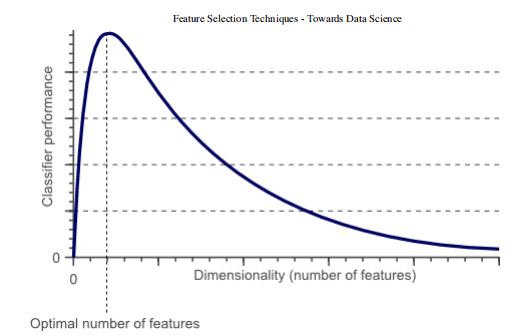1.过滤方法=过滤我们的数据集，只取包含所有相关特征的子集（例如，使用 Pearson 相关的相关矩阵）。

2.遵循过滤方法的相同目标，但使用机器学习模型作为其评估标准（例如，向前/向后/双向/递归特征消除）。我们将一些特征输入机器学习模型，评估它们的性能，然后决定是否添加或删除特征以提高精度。因此，这种方法可以比滤波更精确，但计算成本更高。

3.嵌入方法。与过滤方法一样，嵌入方法也使用机器学习模型。这两种方法的区别在于，嵌入的方法检查 ML 模型的不同训练迭代，然后根据每个特征对 ML 模型训练的贡献程度对每个特征的重要性进行排序。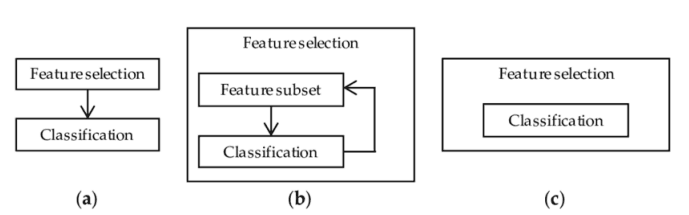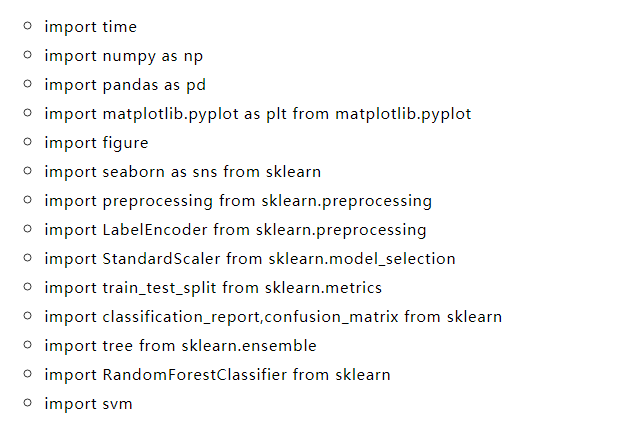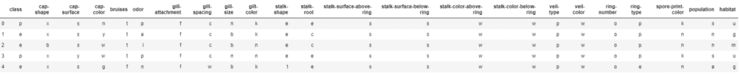X = df.drop(['class'], axis = 1)Y = df['class']X = pd.get_dummies(X, prefix_sep='_')Y = LabelEncoder().fit_transform(Y)
X2 = StandardScaler().fit_transform(X)
X_Train, X_Test, Y_Train, Y_Test = train_test_split(X2, Y, test_size = 0.30,  random_state = 101)

start = time.process_time()trainedforest = RandomForestClassifier(n_estimators=700).fit(X_Train,Y_Train)print(time.process_time() - start)predictionforest = trainedforest.predict(X_Test)print(confusion_matrix(Y_Test,predictionforest))print(classification_report(Y_Test,predictionforest))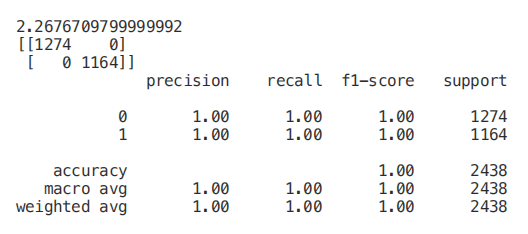figure(num=None, figsize=(20, 22), dpi=80, facecolor='w', edgecolor='k')
feat_importances = pd.Series(trainedforest.feature_importances_, index= X.columns)feat_importances.nlargest(7).plot(kind='barh')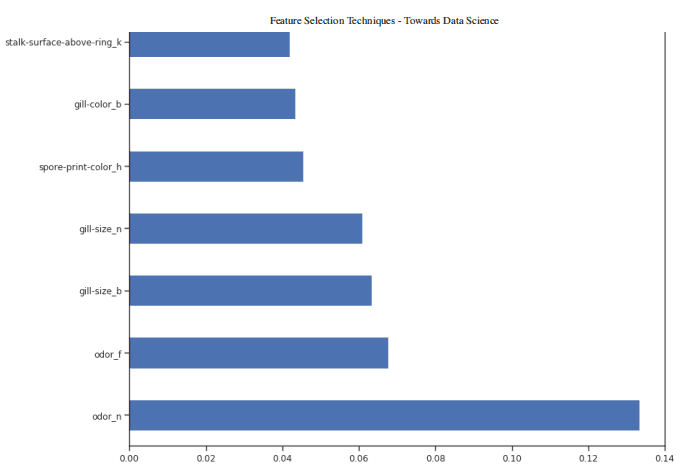X_Reduced = X[['odor_n','odor_f', 'gill-size_n','gill-size_b']]X_Reduced = StandardScaler().fit_transform(X_Reduced)X_Train2, X_Test2, Y_Train2, Y_Test2 = train_test_split(X_Reduced, Y, test_size = 0.30,  random_state = 101)
start = time.process_time()trainedforest = RandomForestClassifier(n_estimators=700).fit(X_Train2,Y_Train2)print(time.process_time() - start)predictionforest = trainedforest.predict(X_Test2)print(confusion_matrix(Y_Test2,predictionforest))print(classification_report(Y_Test2,predictionforest))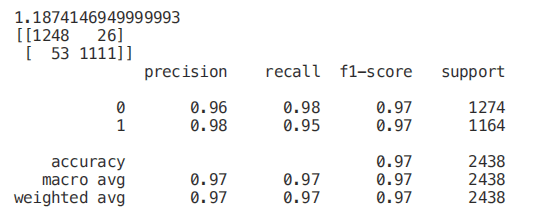start = time.process_time()trainedtree = tree.DecisionTreeClassifier().fit(X_Train, Y_Train)print(time.process_time() - start)predictionstree = trainedtree.predict(X_Test)print(confusion_matrix(Y_Test,predictionstree))print(classification_report(Y_Test,predictionstree))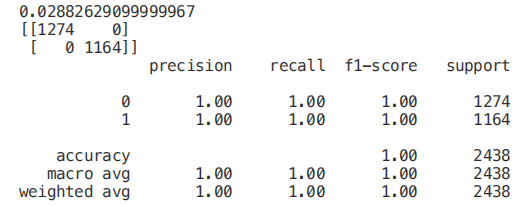import graphvizfrom sklearn.tree import DecisionTreeClassifier, export_graphviz

data = export_graphviz(trainedtree,out_file=None,feature_names= X.columns,        class_names=['edible', 'poisonous'],         filled=True, rounded=True,         max_depth=2,        special_characters=True)graph = graphviz.Source(data)graph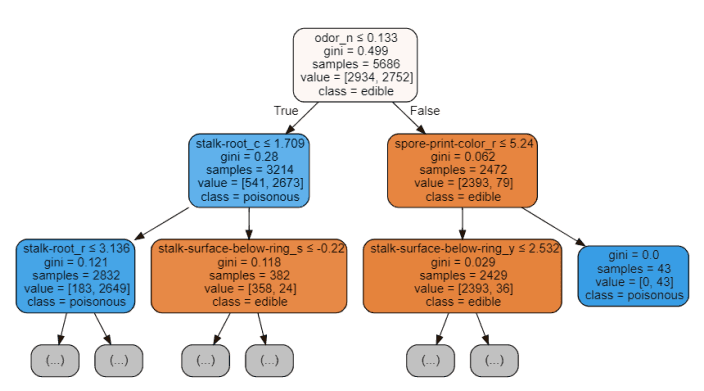from sklearn.feature_selection import RFE
model = RandomForestClassifier(n_estimators=700)rfe = RFE(model, 4)start = time.process_time()RFE_X_Train = rfe.fit_transform(X_Train,Y_Train)RFE_X_Test = rfe.transform(X_Test)rfe = rfe.fit(RFE_X_Train,Y_Train)print(time.process_time() - start)print("Overall Accuracy using RFE: ", rfe.score(RFE_X_Test,Y_Test))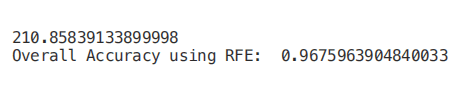SelecFromModel

selectfrommodel 是另一种 scikit 学习方法，可用于特征选择。此方法可用于具有 coef 或 feature 重要性属性的所有不同类型的 scikit 学习模型（拟合后）。与 rfe 相比，selectfrommodel 是一个不太可靠的解决方案。实际上，selectfrommodel 只是根据计算出的阈值（不涉及优化迭代过程）删除不太重要的特性。

ExtratreesClassifier（极端随机树）是基于树的集成分类器，与随机森林方法相比，它可以产生更少的方差（因此减少了过拟合的风险）。随机森林和极随机树的主要区别在于极随机树中节点的采样不需要替换。

from sklearn.ensemble import ExtraTreesClassifierfrom sklearn.feature_selection import SelectFromModel
model = ExtraTreesClassifier()start = time.process_time()model = model.fit(X_Train,Y_Train)model = SelectFromModel(model, prefit=True)print(time.process_time() - start)Selected_X = model.transform(X_Train)
start = time.process_time()trainedforest = RandomForestClassifier(n_estimators=700).fit(Selected_X, Y_Train)print(time.process_time() - start)Selected_X_Test = model.transform(X_Test)predictionforest = trainedforest.predict(Selected_X_Test)print(confusion_matrix(Y_Test,predictionforest))print(classification_report(Y_Test,predictionforest))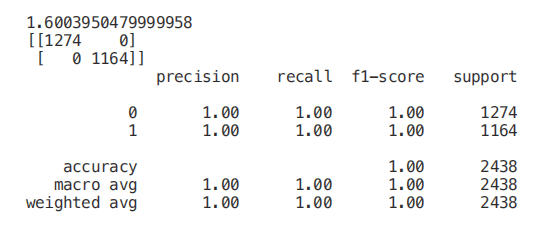• 如果两个特征之间的相关性为 0，则意味着更改这两个特征中的任何一个都不会影响另一个。

• 如果两个特征之间的相关性大于 0，这意味着增加一个特征中的值也会增加另一个特征中的值（相关系数越接近 1，两个不同特征之间的这种联系就越强）。

• 如果两个特征之间的相关性小于 0，这意味着增加一个特征中的值将使减少另一个特征中的值（相关性系数越接近-1，两个不同特征之间的这种关系将越强）。

Numeric_df = pd.DataFrame(X)Numeric_df['Y'] = Ycorr= Numeric_df.corr()corr_y = abs(corr["Y"])highest_corr = corr_y[corr_y >0.5]highest_corr.sort_values(ascending=True)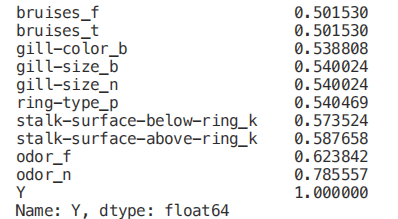figure(num=None, figsize=(12, 10), dpi=80, facecolor='w', edgecolor='k')
corr2 = Numeric_df[['bruises_f' , 'bruises_t' , 'gill-color_b' , 'gill-size_b' ,  'gill-size_n' , 'ring-type_p' , 'stalk-surface-below-ring_k' ,  'stalk-surface-above-ring_k' , 'odor_f', 'odor_n']].corr()
sns.heatmap(corr2, annot=True, fmt=".2g")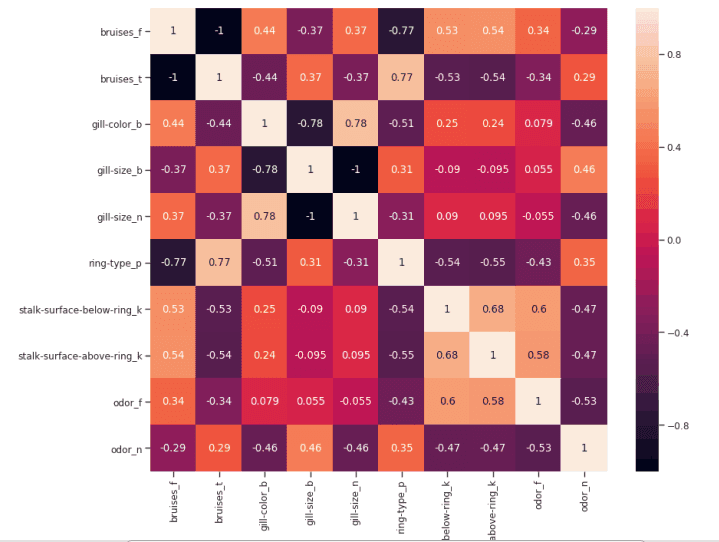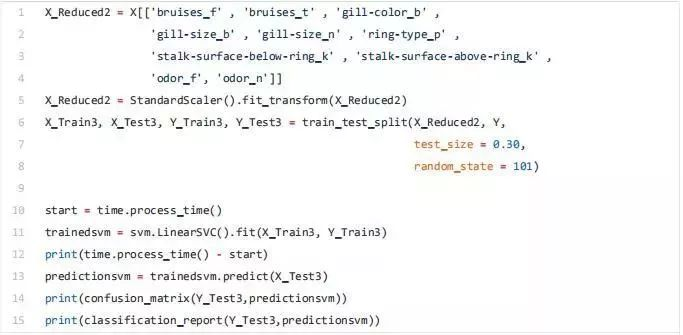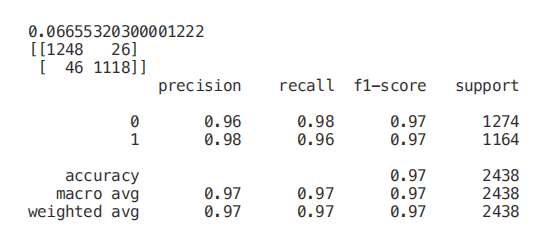• Classification = chi2, f_classif, mutual_info_classif

• Regression = f_regression, mutual_info_regression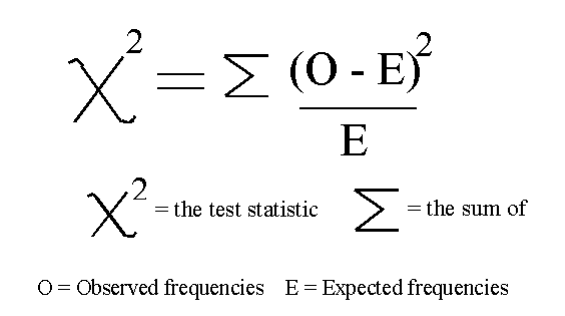from sklearn.feature_selection import SelectKBestfrom sklearn.feature_selection import chi2
min_max_scaler = preprocessing.MinMaxScaler()Scaled_X = min_max_scaler.fit_transform(X2)
X_new = SelectKBest(chi2, k=2).fit_transform(Scaled_X, Y)X_Train3, X_Test3, Y_Train3, Y_Test3 = train_test_split(X_new, Y, test_size = 0.30,  random_state = 101)start = time.process_time()trainedforest = RandomForestClassifier(n_estimators=700).fit(X_Train3,Y_Train3)print(time.process_time() - start)predictionforest = trainedforest.predict(X_Test3)print(confusion_matrix(Y_Test3,predictionforest))print(classification_report(Y_Test3,predictionforest))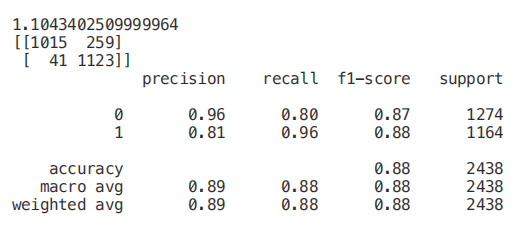from sklearn.linear_model import LassoCV
regr = LassoCV(cv=5, random_state=101)regr.fit(X_Train,Y_Train)print("LassoCV Best Alpha Scored: ", regr.alpha_)print("LassoCV Model Accuracy: ", regr.score(X_Test, Y_Test))model_coef = pd.Series(regr.coef_, index = list(X.columns[:-1]))print("Variables Eliminated: ", str(sum(model_coef == 0)))print("Variables Kept: ", str(sum(model_coef != 0)))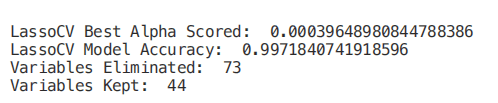figure(num=None, figsize=(12, 10), dpi=80, facecolor='w', edgecolor='k')
top_coef = model_coef.sort_values()top_coef[top_coef != 0].plot(kind = "barh")plt.title("Most Important Features Identified using Lasso (!0)")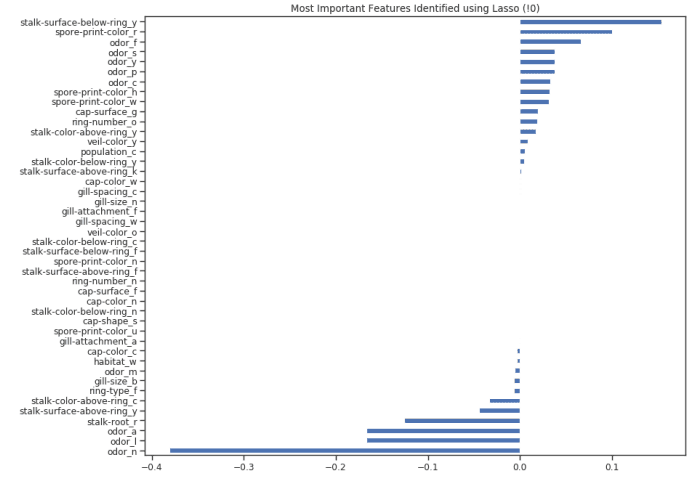via：https://towardsdatascience.com/feature-selection-techniques-1bfab5fe0784©️2019 CSDN 皮肤主题: 大白 设计师: CSDN官方博客